Descriptive Statistics

# 13 Measures of the Spread of the Data

An important characteristic of any set of data is the variation in the data. In some data sets, the data values are concentrated closely near the mean; in other data sets, the data values are more widely spread out from the mean. The most common measure of variation, or spread, is the standard deviation. The standard deviation is a number that measures how far data values are from their mean.

### The standard deviation

• provides a numerical measure of the overall amount of variation in a data set, and
• can be used to determine whether a particular data value is close to or far from the mean.

#### The standard deviation provides a measure of the overall variation in a data set

The standard deviation is always positive or zero. The standard deviation is small when the data are all concentrated close to the mean, exhibiting little variation or spread. The standard deviation is larger when the data values are more spread out from the mean, exhibiting more variation.

Suppose that we are studying the amount of time customers wait in line at the checkout at supermarket A and supermarket B. The average wait time at both supermarkets is five minutes. At supermarket A, the standard deviation for the wait time is two minutes; at supermarket B. The standard deviation for the wait time is four minutes.

Because supermarket B has a higher standard deviation, we know that there is more variation in the wait times at supermarket B. Overall, wait times at supermarket B are more spread out from the average; wait times at supermarket A are more concentrated near the average.

#### Calculating the Standard Deviation

If x is a number, then the difference “x minus the mean” is called its deviation. In a data set, there are as many deviations as there are items in the data set. The deviations are used to calculate the standard deviation. If the numbers belong to a population, in symbols a deviation is xμ. For sample data, in symbols a deviation is x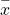.

The procedure to calculate the standard deviation depends on whether the numbers are the entire population or are data from a sample. The calculations are similar, but not identical. Therefore the symbol used to represent the standard deviation depends on whether it is calculated from a population or a sample. The lower case letter s represents the sample standard deviation and the Greek letter σ (sigma, lower case) represents the population standard deviation. If the sample has the same characteristics as the population, then s should be a good estimate of σ.

To calculate the standard deviation, we need to calculate the variance first. The variance is the average of the squares of the deviations (the xvalues for a sample, or the xμ values for a population). The symbol σ2 represents the population variance; the population standard deviation σ is the square root of the population variance. The symbol s2 represents the sample variance; the sample standard deviation s is the square root of the sample variance. You can think of the standard deviation as a special average of the deviations. Formally, the variance is the second moment of the distribution or the first moment around the mean. Remember that the mean is the first moment of the distribution.

If the numbers come from a census of the entire population and not a sample, when we calculate the average of the squared deviations to find the variance, we divide by N, the number of items in the population. If the data are from a sample rather than a population, when we calculate the average of the squared deviations, we divide by n – 1, one less than the number of items in the sample.

#### Formulas for the Sample Standard Deviation

•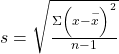or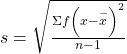or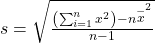• For the sample standard deviation, the denominator is n – 1, that is the sample size minus 1.

#### Formulas for the Population Standard Deviation

•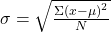or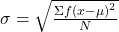or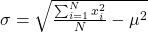• For the population standard deviation, the denominator is N, the number of items in the population.

In these formulas, f represents the frequency with which a value appears. For example, if a value appears once, f is one. If a value appears three times in the data set or population, f is three. Two important observations concerning the variance and standard deviation: the deviations are measured from the mean and the deviations are squared. In principle, the deviations could be measured from any point, however, our interest is measurement from the center weight of the data, what is the “normal” or most usual value of the observation. Later we will be trying to measure the “unusualness” of an observation or a sample mean and thus we need a measure from the mean. The second observation is that the deviations are squared. This does two things, first it makes the deviations all positive and second it changes the units of measurement from that of the mean and the original observations. If the data are weights then the mean is measured in pounds, but the variance is measured in pounds-squared. One reason to use the standard deviation is to return to the original units of measurement by taking the square root of the variance. Further, when the deviations are squared it explodes their value. For example, a deviation of 10 from the mean when squared is 100, but a deviation of 100 from the mean is 10,000. What this does is place great weight on outliers when calculating the variance.

#### Types of Variability in Samples

When trying to study a population, a sample is often used, either for convenience or because it is not possible to access the entire population. Variability is the term used to describe the differences that may occur in these outcomes. Common types of variability include the following:

• Observational or measurement variability
• Natural variability
• Induced variability
• Sample variability

Here are some examples to describe each type of variability.

Example 1: Measurement variabilityMeasurement variability occurs when there are differences in the instruments used to measure or in the people using those instruments. If we are gathering data on how long it takes for a ball to drop from a height by having students measure the time of the drop with a stopwatch, we may experience measurement variability if the two stopwatches used were made by different manufacturers: For example, one stopwatch measures to the nearest second, whereas the other one measures to the nearest tenth of a second. We also may experience measurement variability because two different people are gathering the data. Their reaction times in pressing the button on the stopwatch may differ; thus, the outcomes will vary accordingly. The differences in outcomes may be affected by measurement variability.

Example 2: Natural variabilityNatural variability arises from the differences that naturally occur because members of a population differ from each other. For example, if we have two identical corn plants and we expose both plants to the same amount of water and sunlight, they may still grow at different rates simply because they are two different corn plants. The difference in outcomes may be explained by natural variability.

Example 3: Induced variabilityInduced variability is the counterpart to natural variability; this occurs because we have artificially induced an element of variation (that, by definition, was not present naturally): For example, we assign people to two different groups to study memory, and we induce a variable in one group by limiting the amount of sleep they get. The difference in outcomes may be affected by induced variability.

Example 4: Sample variabilitySample variability occurs when multiple random samples are taken from the same population. For example, if I conduct four surveys of 50 people randomly selected from a given population, the differences in outcomes may be affected by sample variability.

In a fifth grade class, the teacher was interested in the average age and the sample standard deviation of the ages of her students. The following data are the ages for a SAMPLE of n = 20 fifth grade students. The ages are rounded to the nearest half year:

9; 9.5; 9.5; 10; 10; 10; 10; 10.5; 10.5; 10.5; 10.5; 11; 11; 11; 11; 11; 11; 11.5; 11.5; 11.5;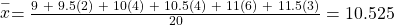The average age is 10.53 years, rounded to two places.

The variance may be calculated by using a table. Then the standard deviation is calculated by taking the square root of the variance. We will explain the parts of the table after calculating s.

Data Freq. Deviations Deviations2 (Freq.)(Deviations2)
x f (x) (x)2 (f)(x)2
9 1 9 – 10.525 = –1.525 (–1.525)2 = 2.325625 1 × 2.325625 = 2.325625
9.5 2 9.5 – 10.525 = –1.025 (–1.025)2 = 1.050625 2 × 1.050625 = 2.101250
10 4 10 – 10.525 = –0.525 (–0.525)2 = 0.275625 4 × 0.275625 = 1.1025
10.5 4 10.5 – 10.525 = –0.025 (–0.025)2 = 0.000625 4 × 0.000625 = 0.0025
11 6 11 – 10.525 = 0.475 (0.475)2 = 0.225625 6 × 0.225625 = 1.35375
11.5 3 11.5 – 10.525 = 0.975 (0.975)2 = 0.950625 3 × 0.950625 = 2.851875
The total is 9.7375

The sample variance, s2, is equal to the sum of the last column (9.7375) divided by the total number of data values minus one (20 – 1):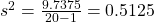The sample standard deviation s is equal to the square root of the sample variance: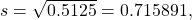which is rounded to two decimal places, s = 0.72.

1. See (Figure)
1. The sample mean = 73.5
2. The sample standard deviation = 17.9
3. The median = 73
4. The first quartile = 61
5. The third quartile = 90
6. IQR = 90 – 61 = 29
Data Frequency Relative frequency Cumulative relative frequency
33 1 0.032 0.032
42 1 0.032 0.064
49 2 0.065 0.129
53 1 0.032 0.161
55 2 0.065 0.226
61 1 0.032 0.258
63 1 0.032 0.29
67 1 0.032 0.322
68 2 0.065 0.387
69 2 0.065 0.452
72 1 0.032 0.484
73 1 0.032 0.516
74 1 0.032 0.548
78 1 0.032 0.580
80 1 0.032 0.612
83 1 0.032 0.644
88 3 0.097 0.741
90 1 0.032 0.773
92 1 0.032 0.805
94 4 0.129 0.934
96 1 0.032 0.966
100 1 0.032 0.998 (Why isn’t this value 1? ANSWER: Rounding)

### Standard deviation of Grouped Frequency Tables

Recall that for grouped data we do not know individual data values, so we cannot describe the typical value of the data with precision. In other words, we cannot find the exact mean, median, or mode. We can, however, determine the best estimate of the measures of center by finding the mean of the grouped data with the formula: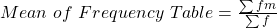where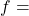interval frequencies and m = interval midpoints.

Just as we could not find the exact mean, neither can we find the exact standard deviation. Remember that standard deviation describes numerically the expected deviation a data value has from the mean. In simple English, the standard deviation allows us to compare how “unusual” individual data is compared to the mean.

Find the standard deviation for the data in (Figure).

Class Frequency, f Midpoint, m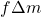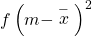0–2 1 1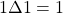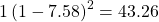3–5 6 4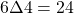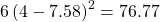6-8 10 7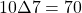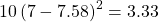9-11 7 10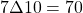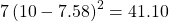12-14 0 13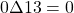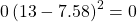26=n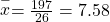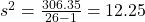For this data set, we have the mean,= 7.58 and the standard deviation, sx = 3.5. This means that a randomly selected data value would be expected to be 3.5 units from the mean. If we look at the first class, we see that the class midpoint is equal to one. This is almost two full standard deviations from the mean since 7.58 – 3.5 – 3.5 = 0.58. While the formula for calculating the standard deviation is not complicated,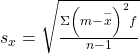where
sx = sample standard deviation,= sample mean, the calculations are tedious. It is usually best to use technology when performing the calculations.

### Comparing Values from Different Data Sets

The standard deviation is useful when comparing data values that come from different data sets. If the data sets have different means and standard deviations, then comparing the data values directly can be misleading.

• For each data value x, calculate how many standard deviations away from its mean the value is.
• Use the formula: x = mean + (#ofSTDEVs)(standard deviation); solve for #ofSTDEVs.
•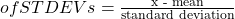• Compare the results of this calculation.

#ofSTDEVs is often called a “z-score”; we can use the symbol z. In symbols, the formulas become:

 Sample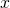=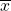+ zs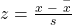Population=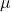+ zσ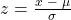Two students, John and Ali, from different high schools, wanted to find out who had the highest GPA when compared to his school. Which student had the highest GPA when compared to his school?

Student GPA School mean GPA School standard deviation
John 2.85 3.0 0.7
Ali 77 80 10

For each student, determine how many standard deviations (#ofSTDEVs) his GPA is away from the average, for his school. Pay careful attention to signs when comparing and interpreting the answer.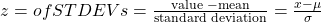For John,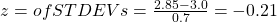For Ali,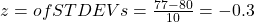John has the better GPA when compared to his school because his GPA is 0.21 standard deviations below his school’s mean while Ali’s GPA is 0.3 standard deviations below his school’s mean.

John’s z-score of –0.21 is higher than Ali’s z-score of –0.3. For GPA, higher values are better, so we conclude that John has the better GPA when compared to his school.

Try It

Two swimmers, Angie and Beth, from different teams, wanted to find out who had the fastest time for the 50 meter freestyle when compared to her team. Which swimmer had the fastest time when compared to her team?

Swimmer Time (seconds) Team mean time Team standard deviation
Angie 26.2 27.2 0.8
Beth 27.3 30.1 1.4

For Angie: z =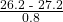= –1.25

For Beth: z =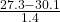= –2

The following lists give a few facts that provide a little more insight into what the standard deviation tells us about the distribution of the data.

For ANY data set, no matter what the distribution of the data is:
• At least 75% of the data is within two standard deviations of the mean.
• At least 89% of the data is within three standard deviations of the mean.
• At least 95% of the data is within 4.5 standard deviations of the mean.
• This is known as Chebyshev’s Rule.
For data having a Normal Distribution, which we will examine in great detail later:
• Approximately 68% of the data is within one standard deviation of the mean.
• Approximately 95% of the data is within two standard deviations of the mean.
• More than 99% of the data is within three standard deviations of the mean.
• This is known as the Empirical Rule.
• It is important to note that this rule only applies when the shape of the distribution of the data is bell-shaped and symmetric. We will learn more about this when studying the “Normal” or “Gaussian” probability distribution in later chapters.

### Coefficient of Variation

Another useful way to compare distributions besides simple comparisons of means or standard deviations is to adjust for differences in the scale of the data being measured. Quite simply, a large variation in data with a large mean is different than the same variation in data with a small mean. To adjust for the scale of the underlying data the Coefficient of Variation (CV) has been developed. Mathematically: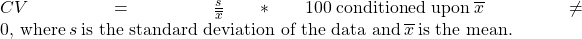We can see that this measures the variability of the underlying data as a percentage of the mean value; the center weight of the data set. This measure is useful in comparing risk where an adjustment is warranted because of differences in scale of two data sets. In effect, the scale is changed to common scale, percentage differences, and allows direct comparison of the two or more magnitudes of variation of different data sets.

### References

Data from Microsoft Bookshelf.

King, Bill.“Graphically Speaking.” Institutional Research, Lake Tahoe Community College. Available online at http://www.ltcc.edu/web/about/institutional-research (accessed April 3, 2013).

### Chapter Review

The standard deviation can help you calculate the spread of data. There are different equations to use if are calculating the standard deviation of a sample or of a population.

• The Standard Deviation allows us to compare individual data or classes to the data set mean numerically.
• s =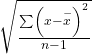or s =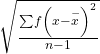is the formula for calculating the standard deviation of a sample. To calculate the standard deviation of a population, we would use the population mean, μ, and the formula σ =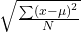or σ =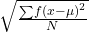.

### Formula Review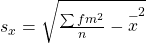where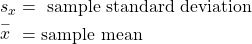Formulas for Sample Standard DeviationororFor the sample standard deviation, the denominator is n – 1, that is the sample size – 1.

Formulas for Population Standard DeviationororFor the population standard deviation, the denominator is N, the number of items in the population.

### Use the following information to answer the next two exercises: The following data are the distances between 20 retail stores and a large distribution center. The distances are in miles. 29; 37; 38; 40; 58; 67; 68; 69; 76; 86; 87; 95; 96; 96; 99; 106; 112; 127; 145; 150Use a graphing calculator or computer to find the standard deviation and round to the nearest tenth.

s = 34.5

Find the value that is one standard deviation below the mean.

Two baseball players, Fredo and Karl, on different teams wanted to find out who had the higher batting average when compared to his team. Which baseball player had the higher batting average when compared to his team?

Baseball player Batting average Team batting average Team standard deviation
Fredo 0.158 0.166 0.012
Karl 0.177 0.189 0.015

For Fredo: z =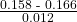= –0.67

For Karl: z =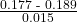= –0.8

Fredo’s z-score of –0.67 is higher than Karl’s z-score of –0.8. For batting average, higher values are better, so Fredo has a better batting average compared to his team.

Use (Figure) to find the value that is three standard deviations:

• above the mean
• below the mean

Find the standard deviation for the following frequency tables using the formula. Check the calculations with the TI 83/84.

Find the standard deviation for the following frequency tables using the formula. Check the calculations with the TI 83/84.

49.5–59.5 2
59.5–69.5 3
69.5–79.5 8
79.5–89.5 12
89.5–99.5 5
2. Daily low temperature Frequency
49.5–59.5 53
59.5–69.5 32
69.5–79.5 15
79.5–89.5 1
89.5–99.5 0
3. Points per game Frequency
49.5–59.5 14
59.5–69.5 32
69.5–79.5 15
79.5–89.5 23
89.5–99.5 2
1.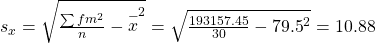2.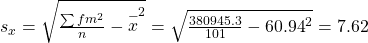3.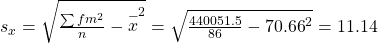### Homework

Use the following information to answer the next nine exercises: The population parameters below describe the full-time equivalent number of students (FTES) each year at Lake Tahoe Community College from 1976–1977 through 2004–2005.

• μ = 1000 FTES
• median = 1,014 FTES
• σ = 474 FTES
• first quartile = 528.5 FTES
• third quartile = 1,447.5 FTES
• n = 29 years

A sample of 11 years is taken. About how many are expected to have a FTES of 1014 or above? Explain how you determined your answer.

The median value is the middle value in the ordered list of data values. The median value of a set of 11 will be the 6th number in order. Six years will have totals at or below the median.

75% of all years have an FTES:

1. at or below: _____
2. at or above: _____

The population standard deviation = _____

474 FTES

What percent of the FTES were from 528.5 to 1447.5? How do you know?

What is the IQR? What does the IQR represent?

919

How many standard deviations away from the mean is the median?

Additional Information: The population FTES for 2005–2006 through 2010–2011 was given in an updated report. The data are reported here.

 Year 2005–06 2006–07 2007–08 2008–09 2009–10 2010–11 Total FTES 1,585 1,690 1,735 1,935 2,021 1,890

Calculate the mean, median, standard deviation, the first quartile, the third quartile and the IQR. Round to one decimal place.

• mean = 1,809.3
• median = 1,812.5
• standard deviation = 151.2
• first quartile = 1,690
• third quartile = 1,935
• IQR = 245

Compare the IQR for the FTES for 1976–77 through 2004–2005 with the IQR for the FTES for 2005-2006 through 2010–2011. Why do you suppose the IQRs are so different?

Hint: Think about the number of years covered by each time period and what happened to higher education during those periods.

Three students were applying to the same graduate school. They came from schools with different grading systems. Which student had the best GPA when compared to other students at his school? Explain how you determined your answer.

Student GPA School Average GPA School Standard Deviation
Thuy 2.7 3.2 0.8
Vichet 87 75 20
Kamala 8.6 8 0.4

A music school has budgeted to purchase three musical instruments. They plan to purchase a piano costing ?3,000, a guitar costing ?550, and a drum set costing ?600. The mean cost for a piano is ?4,000 with a standard deviation of ?2,500. The mean cost for a guitar is ?500 with a standard deviation of ?200. The mean cost for drums is ?700 with a standard deviation of ?100. Which cost is the lowest, when compared to other instruments of the same type? Which cost is the highest when compared to other instruments of the same type. Justify your answer.

For pianos, the cost of the piano is 0.4 standard deviations BELOW the mean. For guitars, the cost of the guitar is 0.25 standard deviations ABOVE the mean. For drums, the cost of the drum set is 1.0 standard deviations BELOW the mean. Of the three, the drums cost the lowest in comparison to the cost of other instruments of the same type. The guitar costs the most in comparison to the cost of other instruments of the same type.

An elementary school class ran one mile with a mean of 11 minutes and a standard deviation of three minutes. Rachel, a student in the class, ran one mile in eight minutes. A junior high school class ran one mile with a mean of nine minutes and a standard deviation of two minutes. Kenji, a student in the class, ran 1 mile in 8.5 minutes. A high school class ran one mile with a mean of seven minutes and a standard deviation of four minutes. Nedda, a student in the class, ran one mile in eight minutes.

1. Why is Kenji considered a better runner than Nedda, even though Nedda ran faster than he?
2. Who is the fastest runner with respect to his or her class? Explain why.

The most obese countries in the world have obesity rates that range from 11.4% to 74.6%. This data is summarized in Table 14.

Percent of population obese Number of countries
11.4–20.45 29
20.45–29.45 13
29.45–38.45 4
38.45–47.45 0
47.45–56.45 2
56.45–65.45 1
65.45–74.45 0
74.45–83.45 1

What is the best estimate of the average obesity percentage for these countries? What is the standard deviation for the listed obesity rates? The United States has an average obesity rate of 33.9%. Is this rate above average or below? How “unusual” is the United States’ obesity rate compared to the average rate? Explain.

•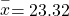• Using the TI 83/84, we obtain a standard deviation of: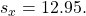• The obesity rate of the United States is 10.58% higher than the average obesity rate.
• Since the standard deviation is 12.95, we see that 23.32 + 12.95 = 36.27 is the obesity percentage that is one standard deviation from the mean. The United States obesity rate is slightly less than one standard deviation from the mean. Therefore, we can assume that the United States, while 34% obese, does not hav e an unusually high percentage of obese people.

(Figure) gives the percent of children under five considered to be underweight.

Percent of underweight children Number of countries
16–21.45 23
21.45–26.9 4
26.9–32.35 9
32.35–37.8 7
37.8–43.25 6
43.25–48.7 1

What is the best estimate for the mean percentage of underweight children? What is the standard deviation? Which interval(s) could be considered unusual? Explain.

### Bringing It Together

Twenty-five randomly selected students were asked the number of movies they watched the previous week. The results are as follows:

# of movies Frequency
0 5
1 9
2 6
3 4
4 1
1. Find the sample mean.
2. Find the approximate sample standard deviation, s.
1. 1.48
2. 1.12

Forty randomly selected students were asked the number of pairs of sneakers they owned. Let X = the number of pairs of sneakers owned. The results are as follows:

X Frequency
1 2
2 5
3 8
4 12
5 12
6 0
7 1
1. Find the sample mean2. Find the sample standard deviation, s
3. Construct a histogram of the data.
4. Complete the columns of the chart.
5. Find the first quartile.
6. Find the median.
7. Find the third quartile.
8. What percent of the students owned at least five pairs?
9. Find the 40th percentile.
10. Find the 90th percentile.
11. Construct a line graph of the data
12. Construct a stemplot of the data

Following are the published weights (in pounds) of all of the team members of the San Francisco 49ers from a previous year.

177; 205; 210; 210; 232; 205; 185; 185; 178; 210; 206; 212; 184; 174; 185; 242; 188; 212; 215; 247; 241; 223; 220; 260; 245; 259; 278; 270; 280; 295; 275; 285; 290; 272; 273; 280; 285; 286; 200; 215; 185; 230; 250; 241; 190; 260; 250; 302; 265; 290; 276; 228; 265

1. Organize the data from smallest to largest value.
2. Find the median.
3. Find the first quartile.
4. Find the third quartile.
5. The middle 50% of the weights are from _______ to _______.
6. If our population were all professional football players, would the above data be a sample of weights or the population of weights? Why?
7. If our population included every team member who ever played for the San Francisco 49ers, would the above data be a sample of weights or the population of weights? Why?
8. Assume the population was the San Francisco 49ers. Find:
1. the population mean, μ.
2. the population standard deviation, σ.
3. the weight that is two standard deviations below the mean.
4. When Steve Young, quarterback, played football, he weighed 205 pounds. How many standard deviations above or below the mean was he?
9. That same year, the mean weight for the Dallas Cowboys was 240.08 pounds with a standard deviation of 44.38 pounds. Emmit Smith weighed in at 209 pounds. With respect to his team, who was lighter, Smith or Young? How did you determine your answer?
1. 174; 177; 178; 184; 185; 185; 185; 185; 188; 190; 200; 205; 205; 206; 210; 210; 210; 212; 212; 215; 215; 220; 223; 228; 230; 232; 241; 241; 242; 245; 247; 250; 250; 259; 260; 260; 265; 265; 270; 272; 273; 275; 276; 278; 280; 280; 285; 285; 286; 290; 290; 295; 302
2. 241
3. 205.5
4. 272.5
5. 205.5, 272.5
6. sample
7. population
1. 236.34
2. 37.50
3. 161.34
4. 0.84 std. dev. below the mean
8. Young

One hundred teachers attended a seminar on mathematical problem solving. The attitudes of a representative sample of 12 of the teachers were measured before and after the seminar. A positive number for change in attitude indicates that a teacher’s attitude toward math became more positive. The 12 change scores are as follows:

3 8–12 05–31–16 5–2

1. What is the mean change score?
2. What is the standard deviation for this population?
3. What is the median change score?
4. Find the change score that is 2.2 standard deviations below the mean.

Refer to (Figure) determine which of the following are true and which are false. Explain your solution to each part in complete sentences.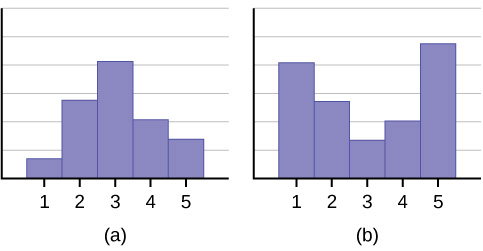1. The medians for both graphs are the same.
2. We cannot determine if any of the means for both graphs is different.
3. The standard deviation for graph b is larger than the standard deviation for graph a.
4. We cannot determine if any of the third quartiles for both graphs is different.
1. True
2. True
3. True
4. False

In a recent issue of the IEEE Spectrum, 84 engineering conferences were announced. Four conferences lasted two days. Thirty-six lasted three days. Eighteen lasted four days. Nineteen lasted five days. Four lasted six days. One lasted seven days. One lasted eight days. One lasted nine days. Let X = the length (in days) of an engineering conference.

1. Organize the data in a chart.
2. Find the median, the first quartile, and the third quartile.
3. Find the 65th percentile.
4. Find the 10th percentile.
5. The middle 50% of the conferences last from _______ days to _______ days.
6. Calculate the sample mean of days of engineering conferences.
7. Calculate the sample standard deviation of days of engineering conferences.
8. Find the mode.
9. If you were planning an engineering conference, which would you choose as the length of the conference: mean; median; or mode? Explain why you made that choice.
10. Give two reasons why you think that three to five days seem to be popular lengths of engineering conferences.

A survey of enrollment at 35 community colleges across the United States yielded the following figures:

6414; 1550; 2109; 9350; 21828; 4300; 5944; 5722; 2825; 2044; 5481; 5200; 5853; 2750; 10012; 6357; 27000; 9414; 7681; 3200; 17500; 9200; 7380; 18314; 6557; 13713; 17768; 7493; 2771; 2861; 1263; 7285; 28165; 5080; 11622

1. Organize the data into a chart with five intervals of equal width. Label the two columns “Enrollment” and “Frequency.”
2. Construct a histogram of the data.
3. If you were to build a new community college, which piece of information would be more valuable: the mode or the mean?
4. Calculate the sample mean.
5. Calculate the sample standard deviation.
6. A school with an enrollment of 8000 would be how many standard deviations away from the mean?
1. Enrollment Frequency
1000-5000 10
5000-10000 16
10000-15000 3
15000-20000 3
20000-25000 1
25000-30000 2
2. Check student’s solution.
3. mode
4. 8628.74
5. 6943.88
6. –0.09

Use the following information to answer the next two exercises.X = the number of days per week that 100 clients use a particular exercise facility.

x Frequency
0 3
1 12
2 33
3 28
4 11
5 9
6 4

The 80th percentile is _____

1. 5
2. 80
3. 3
4. 4

The number that is 1.5 standard deviations BELOW the mean is approximately _____

1. 0.7
2. 4.8
3. –2.8
4. Cannot be determined

a

Suppose that a publisher conducted a survey asking adult consumers the number of fiction paperback books they had purchased in the previous month. The results are summarized in the (Figure).

# of books Freq. Rel. Freq.
0 18
1 24
2 24
3 22
4 15
5 10
7 5
9 1
1. Are there any outliers in the data? Use an appropriate numerical test involving the IQR to identify outliers, if any, and clearly state your conclusion.
2. If a data value is identified as an outlier, what should be done about it?
3. Are any data values further than two standard deviations away from the mean? In some situations, statisticians may use this criteria to identify data values that are unusual, compared to the other data values. (Note that this criteria is most appropriate to use for data that is mound-shaped and symmetric, rather than for skewed data.)
4. Do parts a and c of this problem give the same answer?
5. Examine the shape of the data. Which part, a or c, of this question gives a more appropriate result for this data?
6. Based on the shape of the data which is the most appropriate measure of center for this data: mean, median or mode?

### Key Terms

Standard Deviation
a number that is equal to the square root of the variance and measures how far data values are from their mean; notation: s for sample standard deviation and σ for population standard deviation.
Variance
mean of the squared deviations from the mean, or the square of the standard deviation; for a set of data, a deviation can be represented as xwhere x is a value of the data andis the sample mean. The sample variance is equal to the sum of the squares of the deviations divided by the difference of the sample size and one.